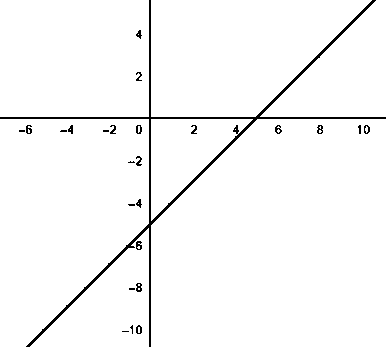Is the given graph of the linear equations in two variables, 5=x-y true or false?

# Is the given graph of the linear equations in two variables, $5=x-y$ true or false?1. A
True
2. B
False

Fill Out the Form for Expert Academic Guidance!l

+91

Live ClassesBooksTest SeriesSelf Learning

Verify OTP Code (required)

### Solution:

It is given, $5=x-y$.
To draw the graph of the equation, we used the plotted point method,
 x y 0 -5 -1 -6 1 -4
Using the above table, the graph can be obtained as:The above graph matches the graph given in the question.
Therefore, the given graph is correct/true.
Therefore, option 1) is correct.

## Related content

 Area of Square Area of Isosceles Triangle Pythagoras Theorem Triangle Formula Perimeter of Triangle Formula Area Formulae Volume of Cone Formula Matrices and Determinants_mathematics Critical Points Solved Examples Type of relations_mathematics+91

Live ClassesBooksTest SeriesSelf Learning

Verify OTP Code (required)